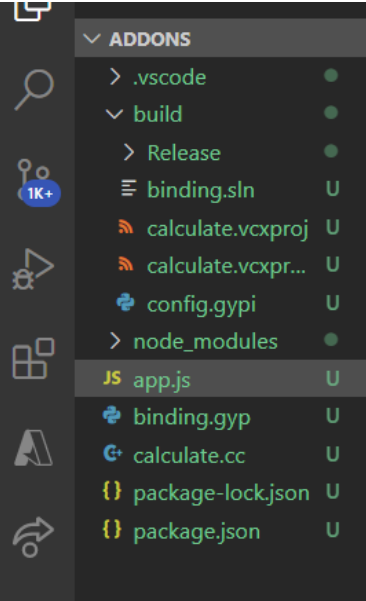Why do we need C++ Addons in Node.js ?

• Last Updated : 20 Jul, 2021

• It can be loaded into Node.js using the require() function and also used just as if they were an ordinary Node.js module.
• It is used primarily to provide an interface between JavaScript running in Node.js and C/C++ libraries.

Why do we need C++ Addons?

• It gives the opportunity to make intensive, parallel, and high-accuracy calculations.
• It also gives the opportunity to use C++ libraries in NodeJS.
• We can integrate a third-party library written in C/C++ and use it directly in NodeJS.
• The performance of C++ is far better on larger values or computation.

Pre-requisites:

• Basic knowledge of Node.
• Node.js installed (version 12+).
• Npm installed (version 6+).

Module Installation: Install the required module using the following command.

npm i -g  node-gyp

Folder Structure: It will look like this.Now create binding.gyp, calculate.cc file with the following code.

Filename: binding.gyp

{
"targets": [
{
"target_name": "calculate", // Name of C++ Addons.
"sources": [ "calculate.cc" ] // Source of C++ file.
}
]
}

calculate.cc

 #include #include   namespace calculate {     using v8:: FunctionCallbackInfo;     using v8 :: Isolate;     using v8 :: Local;     using v8 :: Object;     using v8 :: Number;     using v8 :: Value;       // C++ add function.     void Sum(const FunctionCallbackInfo&args)     {         Isolate* isolate = args.GetIsolate();         int i;         double x = 3.141526, y = 2.718;         for(i=0; i<1000000000; i++)         {             x += y;         }           auto total = Number::New(isolate,x);         args.GetReturnValue().Set(total);     }       // Exports our method     void Initialize(Local

app.js

 // Require addonsconst calculate = require('./build/Release/calculate');  // Javascript add function.function calc() {      // Two variables.    let i, x = 3.141526, y = 2.718;    for (i = 0; i < 1000000000; i++) {        x += y;      }    let total = x;    return total;}  console.time('c++');calculate.calc();console.timeEnd('c++');  console.time('js');calc();  console.timeEnd('js');

Step to run the application: To build and configure, run the following command.

node-gyp configure build
node app.js

Output:

c++: 1.184s
js: 1.207s

My Personal Notes arrow_drop_up